Back to Adaptive Vision Library Lite website

You are here: Start » Basic

# Basic

## Box

Icon Name Description / Applications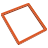BoxCenter

Returns center of a box.BoxCharacteristicPoint

Returns a characteristic point (e.g. the top-left) of a box.BoxCharacteristicPoints

Computes the characteristic points of the box.BoxesBoundingBox

Computes the bounding box of given boxes.BoxesBoundingBox_OrNil

Computes the bounding box of given boxes; returns NIL if the array is empty.BoxIntersection

Computes the common part of two boxes.BoxToBoxDistance

Computes minimal distance between one of the points of the first box with one of the points of the second box.CreateBox

Creates a box.DilateBox

Performs a morphological dilation on a box using box kernel.ErodeBox

Performs a morphological erosion on a box using box kernel.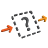RemoveEmptyBoxes

The input boxes which have both dimensions different from zero are copied to the output.

Secures against domain errors caused by empty boxes.ResizeBox

Changes the dimensions of a box.ResizeBox_Delta

Changes the dimensions of a box by adding some values.ResizeBox_Relative

Resizes a box to relatively defined dimensions.SkipEmptyBox

If the input box has both dimensions different from zero, then it is copied to the output; otherwise Nil is returned.

Secures against domain errors caused by empty boxes.SplitBox

Splits a box into two along a direction.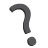TestBoxEmpty

Tests whether the box is empty.TestBoxEqualTo

Tests whether given boxes are equal.TestBoxInBox

Tests whether a box is contained in another one.TestBoxIntersectsWith

Tests whether two boxes have non-empty intersection.TestBoxNotEmpty

Tests whether the box is not empty.TranslateBox

Shifts a box by a discreet vector.

## Format

Icon Name Description / ApplicationsFormatLocationToString

Converts a location to a string of format "(X, Y)".FormatPoint2DToString

Converts a 2D point to a string of format "(X, Y)".FormatPoint3DToString

Converts a 3D point to a string of format "(X, Y, Z)".

## Location

Icon Name Description / Applications
LocationCenter

Returns the center point of a pixel indicated by the provided location.

TranslateLocation

Shifts a Location by a given number of pixels along each axis.

## Matrix

Icon Name Description / Applications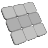AddMatrices

Adds two matrices element by element.CombineMatrices

Combines two matrices horizontally.ConcatenateMatrices

Combines two matrices horizontally.CreateIdentityMatrix

Creates a square matrix with ones on the main diagonal, and zeros elsewhere.CreateUniformMatrix

Creates a matrix with the specified dimensions and filled with a uniform element value.InvertMatrix

Finds the inverse of a square matrix.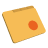LoadMatrix

Loads a serialized Matrix object from an AVDATA file.MultiplyMatrices

Multiplies two matrices element by element.MultiplyMatrixByReal

Multiplies all elements of a matrix by a value.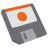SaveMatrix

Saves a serialized Matrix object as AVDATA file.StackMatrices

Combines two matrices vertically.SubtractMatrices

Subtracts two matrices element by element.TransposeMatrix

Swaps rows with columns in a matrix.

## Random

Icon Name Description / Applications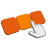CreateRandomArray

Creates array with random values.CreateRandomMatrix

Creates matrix with random values in closed interval.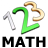RandomInteger

Creates random integer value in given closed interval.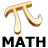RandomReal

Creates random real value in given closed interval.ShuffleArray

Creates an array by setting input array values in the random positions.

## Statistics

Icon Name Description / Applications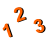Average

Computes the average of an array of real numbers. The array must be not empty.Median

Computes the median of an array of real numbers. The array must be not empty. Optional weights, when supplied, must be positive.Median_InPlace

Computes the median of an array of real numbers. Modifies the input array for the purpose of speed.NthValue

Computes n-th smallest value in an array (0-indexed). The array must be not empty.PearsonCorrelation

Computes Pearson product-moment correlation coefficient. The array must be not empty.Quantile

Computes the specified quantile of an array of real numbers. The array must be not empty.StandardDeviation

Computes the standard deviation of an array of real numbers. The array must be not empty.Variance

Computes the variance of an array of real numbers. The array must be not empty.

## String

Icon Name Description / Applications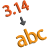FormatDoubleToString

Creates a string from a double number using a proper format.

Useful for preparing a number for display or communication with specific number of fractional digits, sign etc.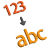FormatIntegerToString

Creates a string from an integer number using a proper format.

Useful for preparing a number for display or communication with specific number of digits, sign etc.FormatRealToString

Creates a string from a real number using a proper format.

Useful for preparing a number for display or communication with specific number of fractional digits, sign etc.LoadText

Loads a text from a file.LoadTextLines

Loads text lines from a file.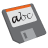SaveText

Saves a text to a file.SaveTextLines

Saves text lines to a file.

## Unit Conversions

Icon Name Description / ApplicationsDegreesToRadiansMillimetresToPixelsPixelsToMillimetresRadiansToDegrees